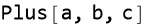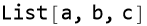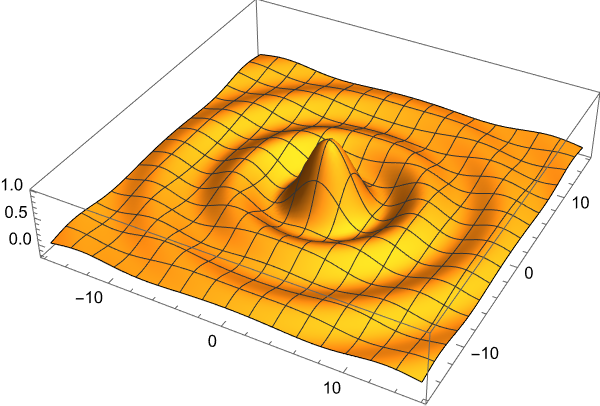```\$Version
```
`13.0.1 for Microsoft Windows (64-bit) (January 28, 2022)`
```FullForm[a+b+c]
``````FullForm[{a,b,c}]
``````neg3=-w // TeXForm
```
\$\$\{-2,-3\}\$\$
```w={2,3}
```
`{2, 3}`
```fun:= Sin[Sqrt[x^2 + y^2]]/Sqrt[x^2 + y^2]
Plot3D[fun, {x, -5*Pi, 5*Pi}, {y, -5*Pi, 5*Pi},
PlotPoints -> 100, BoxRatios -> {1, 1, 0.2},
PlotRange -> All]
``````\$Version
```
`13.0.1 for Microsoft Windows (64-bit) (January 28, 2022)`